# R: ANOVA (Analysis of Variance)

Performing an ANOVA is a standard statistical test to determine if there is a significant difference between multiple sets of data. In this lesson, we will be testing the readings of 4 sensors over the span of a year.

`library(HH)`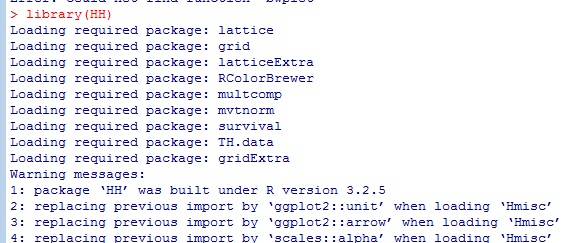Let’s get the data into R

``` mydata <- read.csv(file.choose())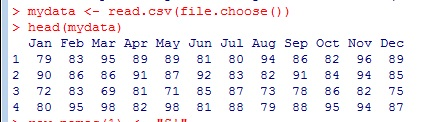Let’s make our data set a bit more user friendly by giving our sensors names (S1,S2,S3,S4)

```row.names(mydata) <- c("S1","S2","S3","S4")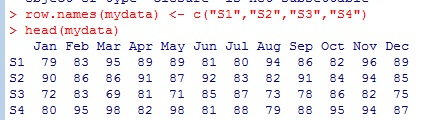Now, our data is in cross tab format. Great for human reading, but not for machine. We need to adjust our data a little.

```#transpose the data
mydataT <- t(mydata)
mydataT```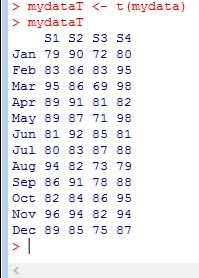Now we need to stack the data. Before we can do that though, we need to convert it from a matrix to a dataframe

`mydataDF <- as.data.frame(mydataT)`

Now let’s stack() our data

```myData1 <- stack(mydataDF)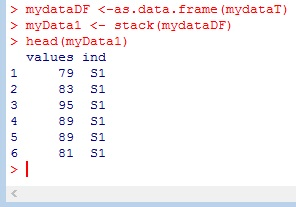stack() makes your data more computer readable. Your column names are turned into elements in a column called “ind”

## Now let’s test our data

I know there were a lot of steps getting here, but I want to assure you that your data will never come in the format you need it in. You will always have to do some sort of manipulation to get it in the format you need.

### Box plot (box-whisker plot)

Box plots are a great way to visualize multiple data sets to see how they compare.

`bwplot(values ~ ind, data = myData1, pch="|")`Take a look at S3, look at how offset it looks – The median doesn’t even cross the 1-3 quartiles of S1,S2, or S4But is this difference significant?

What do I mean by significant? Well, the easiest way for me to explain significance in this case is – can the difference shown above be repeated by random chance? If I took another sampling of the readings would this difference be present or would maybe a difference sensor be out of range?

We use a test called ANOVA (Analysis of Variance) to find out:

### ANOVA (one way)

The code in this case is simple enough

```myData1.fix.aov <- aov(values ~ ind, data=myData1)
anova(myData1.fix.aov)```

It is the results I want to spend a moment onDf of ind= 3 – degrees of freedom – calculated by (4 Sensors – 1)

Df Residuals =44 – this is calculated by total row 48 – 4(the 4 sensors used above)

Sum Sq and Mean Sq = Sum of Squares and Mean Sum of Squares. I am not going to go into the math here, but these two values are calculated comparing the means between our 4 sensors.

You use the Sum Sq and Mean Sq to calculate the F value. You then go find an F chart like the one here: link

You will look up the F ratio (3,44) 3 across, 44 down. These numbers come from the Df( degrees of freedom column). If you look on the table, you will see an F value of 2.82. Our value is 7.4195 WAYYY more than 2.82. This means our ANOVA test proves significance.

It should be noted that the ANOVA test does not tell which sensor is the one out of range. In fact, you could have one or two sensors out of range. We do not know yet. All we know is that something is significantly different.

### p-value

Now I know there are some Stat savvy people reading this who are just dying for me to point out the obvious.The value circled above is your p value. Most standard significance tests use 0.1 or 0.05 as a value to shoot for. If your p-value comes under these values, you have something. Our p-value is 0.0003984 – way under. We definitely found proof of variance.

### mean-mean comparison

Now let’s see what values are different. To do this, we can use a mean-mean comparison chart.

```model.tables(myData1.fix.aov, "means")
myData1.fix.mmc <- mmc(myData1.fix.aov, linfct = mcp(ind = "Tukey"))
myData1.fix.mmc
mmcplot(myData1.fix.mmc, style = "both")```

You can review the Tukey values belowBut I prefer just looking at the chart. To get a quick understanding of how to read this chart, look at the vertical dotted line down the center of the chart. This is the zero line. Now look at the horizontal lines. All the black ones cross the zero line – they are within range of each other.

Look at the red lines now. These are our lines that include S3. It is the means comparisons with S3 that don’t cross the zero line.

This tells you that S3 is the sensor out of range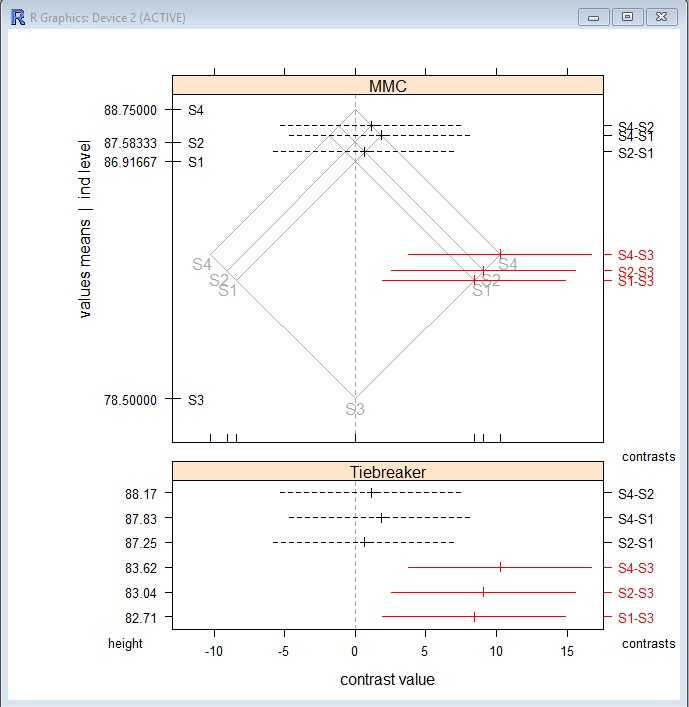Looking back at our box-whisker plot, this really isn’t a surprise### HOV – Brown Forsyth

Finally to confirm our one-way Anova, we have one more test to pass. That is the the homogeneity of variance (Brown Forsyth) test.

In order for a F-test to be valid, the group variances within data must be equal.

```hovBF(values ~ ind, data=myData1)
hovplotBF(values ~ ind, data=myData1)```

Again, I am not going to go into the math, just show you how to read it.

the HOV plot runs difference measures of the spreads of the variances from each sensor from the group median. As you can see in the middle and right plots, the boxes all overlap each other. In a failed HOV, one of the medians in the right plot would not over lap the other 3 boxes.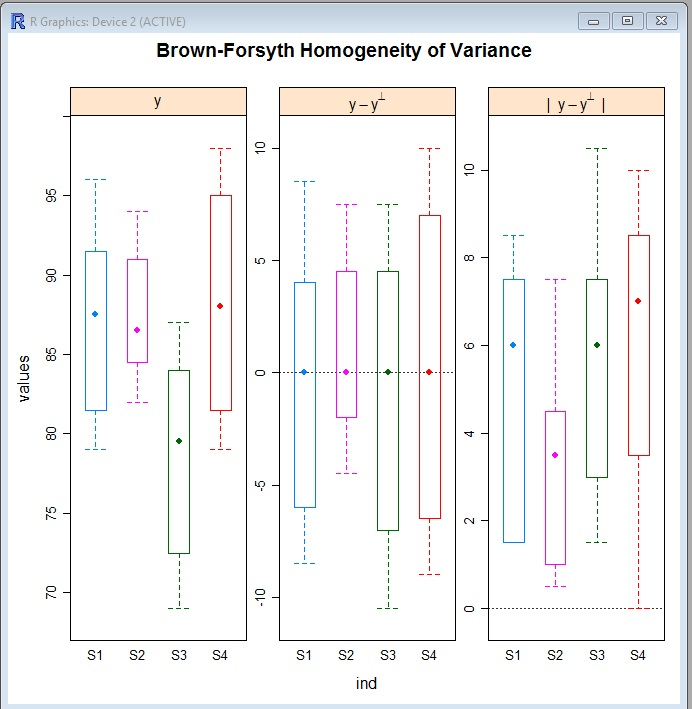In an HOV, looking at our p-value is once again the quickest approach. The p-value here is 0.14 – well above normal significance levels of 0.10 and 0.05.

So, this means there is no reason to disclaim our F-test.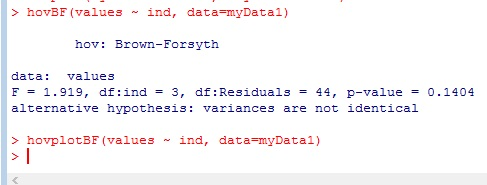So to wrap up everything above, you want a low P value for your F-test and a high P-value for your HOV.

Sorry if you head is spinning a little here. The goal of my site is to go light on the math and show you how to let the computer do the work.

## The Code

```library(HH)

#get data

#name rows
row.names(mydata) <- c("S1","S2","S3","S4")

#transpose data
mydataT <- t(mydata)

#change to dataframe

#box whisker plot
bwplot(values ~ ind, data=myData1)

#anova
myData1.fix.aov <- aov(values ~ ind, data=myData1)
anova(myData1.fix.aov)

#mmc
model.tables(myData1.fix.aov, "means")
myData1.fix.mmc <- mmc(myData1.fix.aov, linfct = mcp(ind = "Tukey"))
myData1.fix.mmc
mmcplot(myData1.fix.mmc, style = "both")

# homogeneity of variance – Brown Forsyth
hovBF(values ~ ind, data=myData1)
hovplotBF(values ~ ind, data=myData1)```

## One thought on “R: ANOVA (Analysis of Variance)”

1.gwlxxqobj@gmail.com

My bro saved this webpage for me and I have been going through it for the past couple hours. This is really going to help me and my classmates for our class project. By the way, I enjoy the way you write.